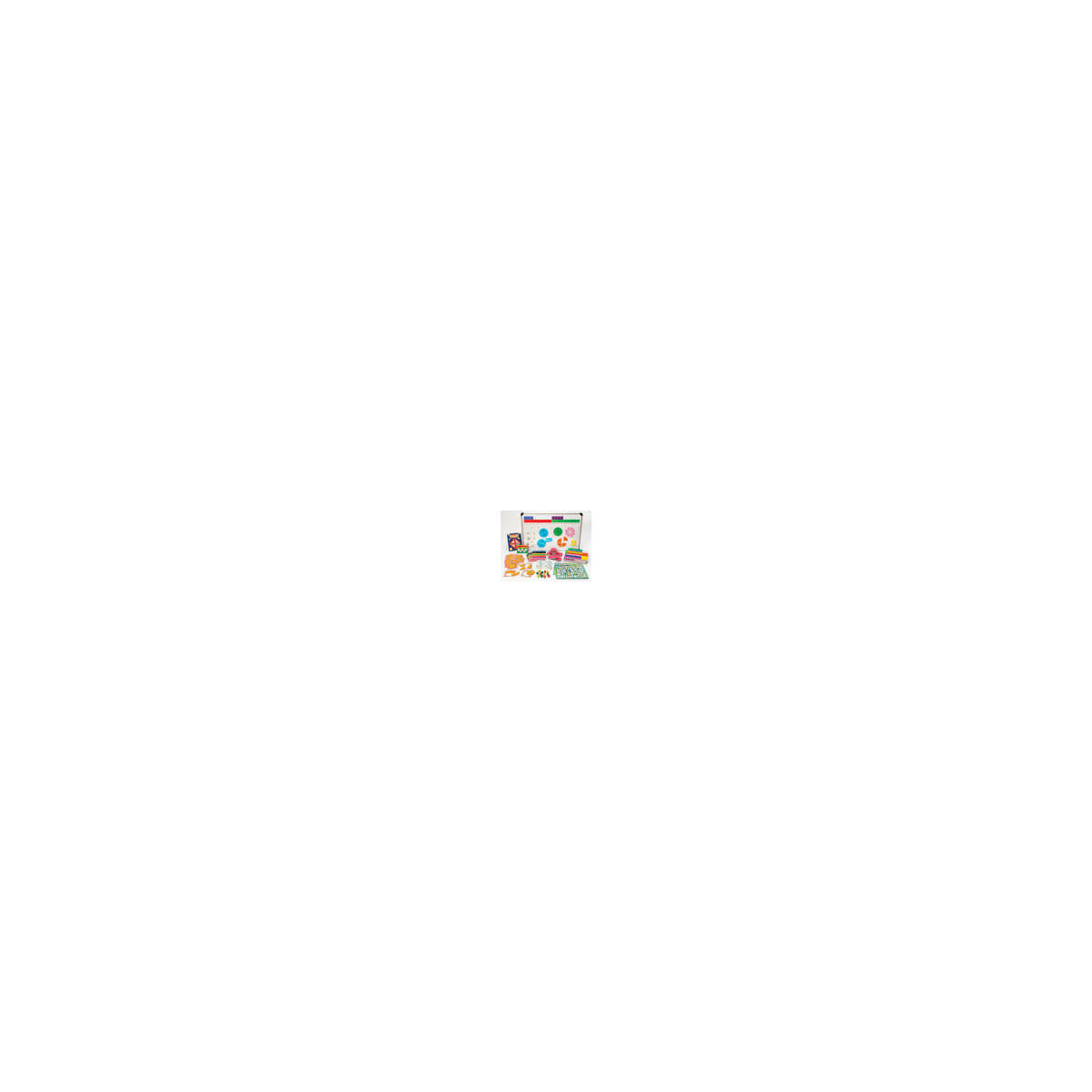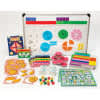•# Elementary Fraction Kit

Product Number: DD-211382
This elementary kit provides students with concrete and symbolic representations of fractions and helps them understand the c...
Quantity

This elementary kit provides students with concrete and symbolic representations of fractions and helps them understand the challenging concepts of fractions, fractional relationships, and equivalency. Includes eight sets each of plastic Fraction Circles, Fraction Squares, and Fraction Tiles; one set each of overhead Fraction Circles, Squares, and Tiles; Fraction Dominoes and Equivalence Dominoes; Fraction Lotto; Fraction Flip Books and Activity Cards; Percent, Decimal, Fraction Flip Stand; 24 Fraction Dice; and Write-On/Wipe-Off Mats
DD-211382